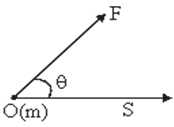CLAT  >  Work, Power and Energy - Physics

# Work, Power and Energy - Physics - Notes | Study Current Affairs & General Knowledge - CLAT

 Table of contentsWorkNegative Work Physics:Zero Work Physics :Law of Conservation of EnergyEinstein Relation1 Crore+ students have signed up on EduRev. Have you?

Work

• Whenever a force acting on a body displaces it, work is said to be done.
• If a force F acting of the body displaces it by a distance s in the direction of force , work done is given by,
W = F.s (or)

W = |F||s| cosθAngle between Force and Displacement

• If θ < 90°, work done is positive and if θ > 90° it is negative.
•  Whenever a force acting on a body displaces it, work is said to be done.
• If a force F acting of the body displaces it by a distance s in the direction of force , work done is given by,
W = F.s (or)

W = |F||s| cosθ

• If θ < 90°, work done is positive and if θ > 90° it is negative.

Examples:

• When body falls freely under gravity, θ = 0, and work done is positive.
• When a lawn roller is pulled by applying a force along the handle at an acute angle, work done by applied force is (+) ve.
• When a spring is stretched or when a gas filled in a cylinder filled with moving piston is allowed to expand, work done is positive.
Negative Work Physics: If θ is obtuse (θ > 90°), work done is negative.
• Examples :
• When a body is thrown up, its motion is opposed by gravity; θ between F and s is 180°.
• When a body is moved over a rough horizontal surface, the motion is opposed by the force of friction.
• Work done by the friction force is negative.
• When brakes are applied to a moving vehicle.
• When a positive charge is moved closer to another positive charge.
Zero Work Physics : If θ is right angle (θ = 90°), work done is zero.

Examples :

• When we fail to move a heavy stone because s > = 0.
• Coollie carrying some load on his head on a horizontal platform θ = 90°.
• Person carrying a briefcase moves on a horizontal road.
• The total capacity of doing work by a body is called its energy.
• It is a scalar quantity.
• Its unit is joule.
Kinetic energy - the energy possessed by a body by its motion is called its kinetic energy.
• K=1/2 mvjoule
Potential energy- it is the energy stored in a body by virtue of its position of state.
• U= mgh
Law of Conservation of Energy
• It states that the total energy remains constant in any process i.e., it may neither be created nor destroyed.
• KE + PE + other forms of energy = constant
• If conservative forces are acting on a body, then,
• KE + PE = constant i.e., the loss of PE is equal to the gain in KE.
Einstein Relation
• According to Einstein, mass can be converted into energy and energy can be converted into mass. One can be obtained at the cost of the other.
• The equivalence between mass and energy is expressed in terms of Einstein relation:
• E = mc²
• Where m is the mass that disappears, E is the energy that appears and c is the velocity of light in vacuum (c = 3 x 108; m/s).
• The rate of doing work by a body is called its power.
• If an agent does work W in time t then average power is,
• P = W/t = F.s/t = F.v = |F||v|cosθ
• It is a scalar quantity.
• SI units of power is watt and is also measured in horse power.
• 1 HP = 746 W (watt)

The document Work, Power and Energy - Physics - Notes | Study Current Affairs & General Knowledge - CLAT is a part of the CLAT Course Current Affairs & General Knowledge.
All you need of CLAT at this link: CLAT

## Current Affairs & General Knowledge

135 videos|627 docs|35 tests
 Use Code STAYHOME200 and get INR 200 additional OFF

## Current Affairs & General Knowledge

135 videos|627 docs|35 tests

Track your progress, build streaks, highlight & save important lessons and more!

,

,

,

,

,

,

,

,

,

,

,

,

,

,

,

,

,

,

,

,

,

,

,

,

;